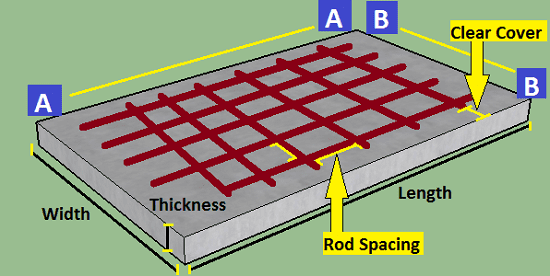# Rebar Calculator for Slab

## Rebar Calculator for Slab## How to use Rebar Calculator for Slab?

• Input all the dimensions in the input section of the above calculator.
• Select the unit of dimension like meter, feet inch, etc.
• Provide the required spacing and diameter of the rebar.
• Input the number of mesh in slab rebar.
• Click on Calculate button to get the total weight of the steel rod in the slab.
• Enjoy the Rebar Calculator for Slab.

Standard Data Taken

The density of Steel Rod = 7850 Kg/m3
1 meter = 3.28084 feet

Rebar calculator for slab helps you find the total weight of steel rod required to construct the slab. Only you have to provide a proper dimension of slab and steel rods.

## How to calculate rebar per square foot?

At first, you have calculate the total weight of the steel rod in a given total area. Now, convert the area into the square feet.

Finally divide the weight of steel bar by total area to get the value of rebar per square foot.

Example, We have a slab of length = 5 meter, width = 3 meter and Total weight of steel required = 750 Kg.

Solution, Area of Slab = 5 x 3 = 15 meter Square = 161.459 Square Foot.

Therefore, Rebar per square foot = 750/161.459 = 4.645 Kg/Foot

## How to calculate rebar quantity?

How to calculate weight of steel bar dia 8mm, 10mm, 12mm, 16mm- Excell

## How to calculate the rebar quantity in Slab?

Calculate weight of steel bar | Quantity of steel bar in circular slab

Share On:"Structural Engineer" with over 5 years of experience in estimation, structural design, and surveying. I am passionate about using his skills to create safe and sustainable structures. I am also a keen writer, and I enjoy sharing my knowledge and experiences with others.